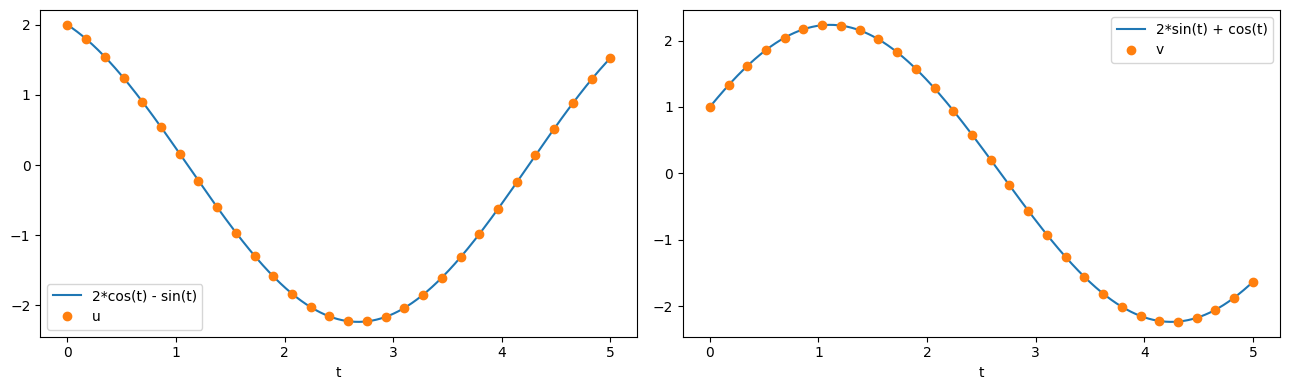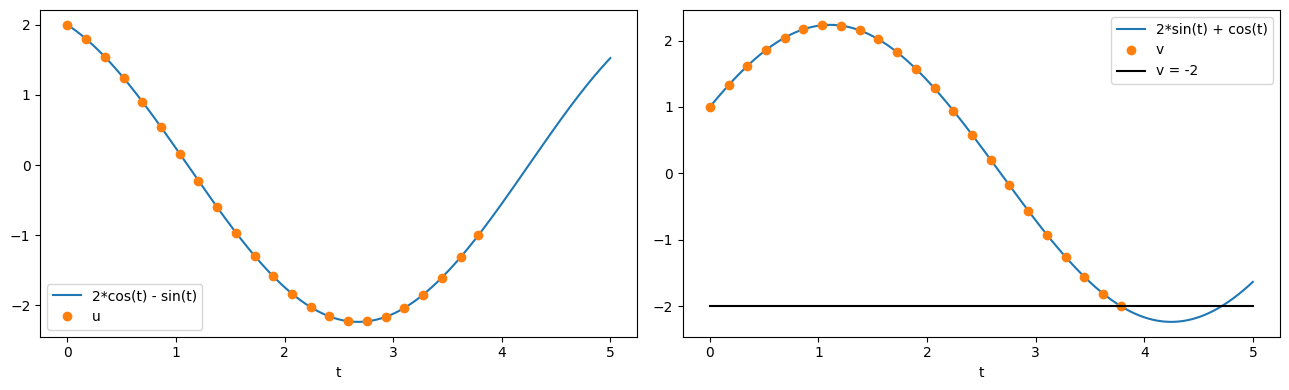Tip

An interactive online version of this notebook is available, which can be accessed via# ODE solver#

In this notebook, we show some examples of solving an ODE model. For the purposes of this example, we use the Scipy solver, but the syntax remains the same for other solvers

:

%pip install "pybamm[plot,cite]" -q    # install PyBaMM if it is not installed
import pybamm
import numpy as np
import os
import matplotlib.pyplot as plt
os.chdir(pybamm.__path__+'/..')

WARNING: You are using pip version 22.0.4; however, version 22.3.1 is available.
You should consider upgrading via the '/home/mrobins/git/PyBaMM/env/bin/python -m pip install --upgrade pip' command.
Note: you may need to restart the kernel to use updated packages.


## Integrating ODEs#

In PyBaMM, a model is solved by calling a solver with solve. This sets up the model to be solved, and then calls the method _integrate, which is specific to each solver. We begin by setting up and discretising a model

:

# Create model
model = pybamm.BaseModel()
u = pybamm.Variable("u")
v = pybamm.Variable("v")
model.rhs = {u: -v, v: u}
model.initial_conditions = {u: 2, v: 1}
model.variables = {"u": u, "v": v}

# Discretise using default discretisation
disc = pybamm.Discretisation()
disc.process_model(model);


Now the model can be solved by calling solver.solve with a specific time vector at which to evaluate the solution

:

# Solve ########################
t_eval = np.linspace(0, 5, 30)
ode_solver = pybamm.ScipySolver()
solution = ode_solver.solve(model, t_eval)
################################

# Extract u and v
t_sol = solution.t
u = solution["u"]
v = solution["v"]

# Plot
t_fine = np.linspace(0,t_eval[-1],1000)

fig, (ax1, ax2) = plt.subplots(1, 2, figsize=(13,4))
ax1.plot(t_fine, 2 * np.cos(t_fine) - np.sin(t_fine), t_sol, u(t_sol), "o")
ax1.set_xlabel("t")
ax1.legend(["2*cos(t) - sin(t)", "u"], loc="best")

ax2.plot(t_fine, 2 * np.sin(t_fine) + np.cos(t_fine), t_sol, v(t_sol), "o")
ax2.set_xlabel("t")
ax2.legend(["2*sin(t) + cos(t)", "v"], loc="best")

plt.tight_layout()
plt.show()Note that, where possible, the solver makes use of the mass matrix and jacobian for the model. However, the discretisation or solver will have created the mass matrix and jacobian algorithmically, using the expression tree, so we do not need to calculate and input these manually.

The solution terminates at the final simulation time:

:

solution.termination

:

'final time'


### Events#

It is possible to specify events at which a solution should terminate. This is done by adding events to the model.events dictionary. In the following example, we solve the same model as before but add a termination event when v=-2.

:

# Create model
model = pybamm.BaseModel()
u = pybamm.Variable("u")
v = pybamm.Variable("v")
model.rhs = {u: -v, v: u}
model.initial_conditions = {u: 2, v: 1}
model.events.append(pybamm.Event('v=-2', v + 2)) # New termination event
model.variables = {"u": u, "v": v}

# Discretise using default discretisation
disc = pybamm.Discretisation()
disc.process_model(model)

# Solve ########################
t_eval = np.linspace(0, 5, 30)
ode_solver = pybamm.ScipySolver()
solution = ode_solver.solve(model, t_eval)
################################

# Extract u and v
t_sol = solution.t
u = solution["u"]
v = solution["v"]

# Plot
t_fine = np.linspace(0,t_eval[-1],1000)

fig, (ax1, ax2) = plt.subplots(1, 2, figsize=(13,4))
ax1.plot(t_fine, 2 * np.cos(t_fine) - np.sin(t_fine), t_sol, u(t_sol), "o")
ax1.set_xlabel("t")
ax1.legend(["2*cos(t) - sin(t)", "u"], loc="best")

ax2.plot(t_fine, 2 * np.sin(t_fine) + np.cos(t_fine), t_sol, v(t_sol), "o", t_fine, -2 * np.ones_like(t_fine), "k")
ax2.set_xlabel("t")
ax2.legend(["2*sin(t) + cos(t)", "v", "v = -2"], loc="best")

plt.tight_layout()
plt.show()Now the solution terminates because the event has been reached

:

solution.termination

:

'event: v=-2'

:

print("event time: ", solution.t_event, "\nevent state", solution.y_event.flatten())

event time:  [3.78510677]
event state [-0.99995359 -2.        ]